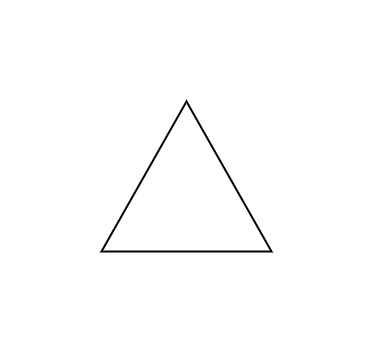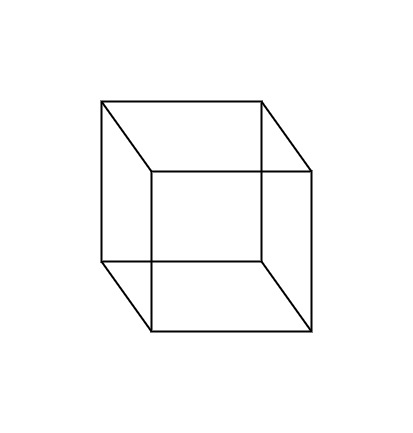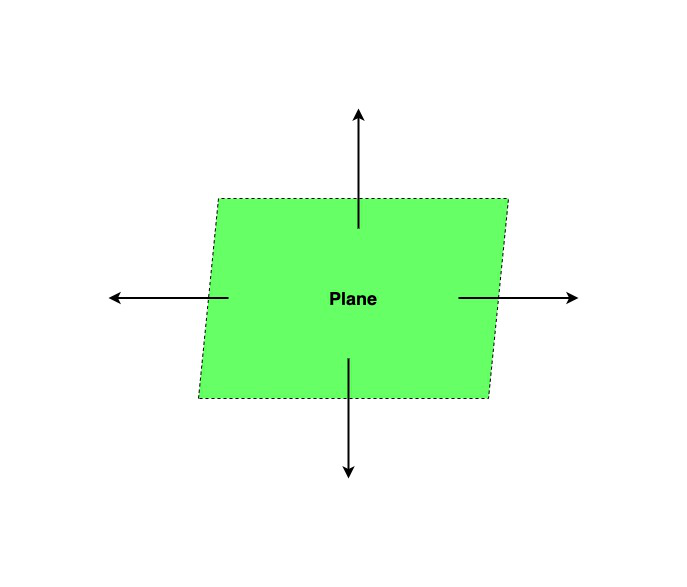GeeksforGeeks App
Open AppBrowser
Continue

# How many dimensions does a plane have?

Geometry is one of the oldest parts of mathematics which deals with lines, points, angles, vertices, etc. They are the visual study of shapes and sizes. It can also be defined as the branch of mathematics that deals with spatial relationships. The given article is focused on a detailed study about plane surfaces. It comprises the definition of planes, their properties with a short description of the dimensions of a plane surface.

2D shapes

Those shapes which have only two dimensions such as height and width are known as 2D shapes. 2D shapes are flat and closed shapes, created by using line segments that are connected to each other. They do not have thickness. Rectangle and circle are some examples of it. Except for circles, all the 2D shapes have vertices, sides, and angles.3D shapes

The multidimensional shapes which have three dimensions such as height, depth, and width, and can be held physically are called 3D shapes. They are also closed shapes and have faces, edges, and vertices. Sphere and prism are examples of 3D shapes.Plane

A plane can be defined as a flat surface or two-dimensional closed figure which has no thickness and curvature. It extends to infinity in all directions. The only dimension a plane is concerned with is its length and breadth. So, in general, to determine a plane we can take anyone’s side or face of a three-dimensional shape such as a side of a cube, a side of a cuboid, circulate a portion of the cone, etc. Some of the real-life examples of planes we can observe around us are board, floor, wall, side of a square box, etc. In a plane, the coordinates represent the location of the points on the plane. Some of the planes are:Properties of the plane:

• In three-dimensional space, two planes are either parallel to each other or intersecting in a specific line.
• If two planes perpendicular to the same line are present then they must be parallel to each other.
• If there are two lines that are perpendicular to the same plane, then they must be parallel to each other.
• The planes that never intersect each other are known as parallel planes. For example, the opposite walls of the cube.
• When two planes intersect each other along a line and are not parallel to each other is known as Intersecting planes. Planes cannot intersect more than one line. For example, the connecting walls of the cube.

### How many dimensions does a plane have?

Solution:

Planes are two-dimensional surfaces. These two dimensions’ length and breadth exceed infinity which means they have no end. The plane does not possess the third dimension which is thickness. As the planes are used to plot shapes on it. The shapes plotted on these plane surfaces are also two-dimensional shapes that have dimensions like length and breadth. They do not possess thickness.

### Sample Questions

Question 1. Give some real-life examples of planes.

Solution:

Some of the real-life examples of planes are walls, floor, paper sheets,etc.

Question 2. How many basic flat shapes are there which can be plotted on a plane surface?

Solution:

There are 5 basic flat shapes as triangle, square, rectangle, circle, and oval can be plotted on a plane surface.

Question 3. How many dimensions are there?

Solution:

There are three dimensions length, breadth, and height.

Question 4. How many dimensions does a plane have?

Solution:

Basically, the plane has two dimensions that are length and breadth.

Question 5. Which 2D shape doesn’t consist of any sides, vertices, and angles?

Solution:

Circle is the only 2D shape that doesn’t consist of any sides, vertices, and angles.

My Personal Notes arrow_drop_up
Related Tutorials